+ Text Only Site
+ Non-Flash Version
+ Contact Glenn##Propulsion System Analysis Answers1. Why do different aircraft have different types of propulsion systems?

Different propulsion systems produce thrust in different ways. Some ways are more fuel efficient than others. Depending on the mission of the aircraft, a certain propulsion system is better suited for a given aircraft. High thrust aircraft typically have worse fuel economy than low thrust (cruise) aircraft.

2. When would you use a ramjet on an airplane?

3. Find the Mach number for a subsonic airplane flying at 650 mph.
 M = Mach M = V/A V = Air Velocity M =.85 A = 762 mph (speed of sound) V = 650 mph
4. Find the Mach number for a subsonic airplane flying at 525 mph.

M = 525 mph/762 mph = .69

5. Find the Mach number for a suubsonic airplane flying at 725 mph.

M = 725 mph/762 mph = .95

6. Find the velocity of a subsonic airplane flying at Mach number of .65.
 M =V/A M = Mach .65 = V/762 V = Air Velocity V = 495.3 mph A = 762 mph. (speed of sound)
7. Find the velocity of a subsonic airplane flying at Mach number of .80.

M = V/A
.8 = V/762
V = 609.6 mph

8. Find the velocity of a supersonic airplane flying at Mach number of 1.5.

M = V/A
V = M * A = 1.5 * 762
V = 1143 mph

9. Using the interactive Atmosphere Calculator, find the speed of sound, pressure and temperature at the following altitudes using English Units.
 ALTITUDE SOUND SPEED PRESSURE TEMPERATURE 1,000 ft. 1113 14.17 55 5,000 ft. 1097 12.23 41 10,000 ft. 1077 10.11 23 20,000 ft. 1037 6.76 -12 25,000 ft. 1016 5.46 -30 30,000 ft. 995 4.37 -47

10. Produce three graphs from the data recorded above, showing speed of sound, pressure, and temperature versus altitude.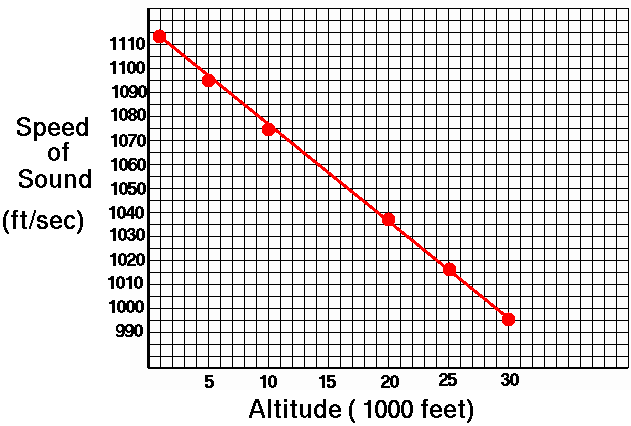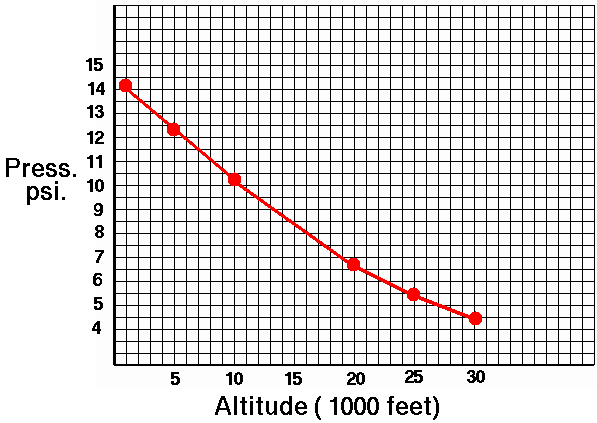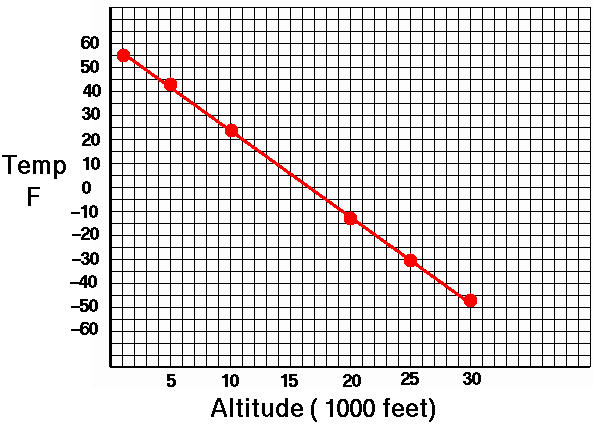11. What effect does altitude have on the speed of sound?

The speed of sound decreases with increasing altitude.

12. What effect does altitude have on pressure?

The atmospheric pressure decreases with increasing altitude.

13. What effect does altitude have on temperature?

The atmospheric temperature decreases with increasing altitude.

14. Flying at a constant speed of 600 feet per second, find the speed of sound and the Mach number at the following altitudes.
 ALTITUDE SOUND SPEED MACH NUMBER 1,000 ft. 1113 .539 10,000 ft. 1077 .556 20,000 ft. 1037 .578 30,000 ft. 995 .602
15. Graph the changes in Mach Number at the different altitudes.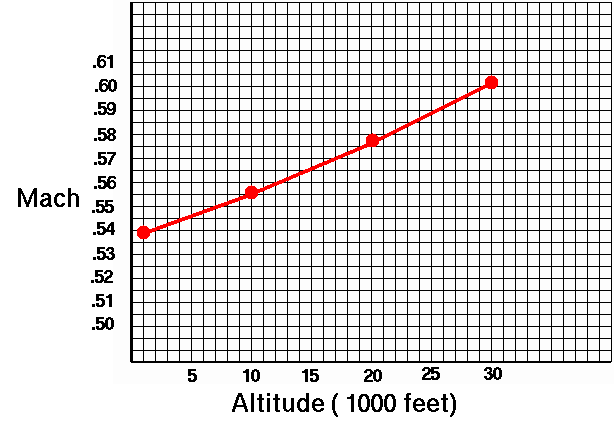16. How does the change in altitude affect Mach Number when flying at a constant speed?

The Mach number increases with increasing altitude for the same speed.

17. The thrust of a jet engine is also affected by changes in altitude. Calculate the thrust of a Pratt & Whitney JT8D-17 jet engine (17,000 pounds at sea level) at different altitudes using the temperature and pressure results from Question 9, and the following equation:

 F = thrust at altitude F sl = sea level static thrust at takeoff (17,000 pounds) P = static pressure at altitude P sl = sea level static pressure (14.7 psi) F = F sl x P/P sl x sqrt(T sl/T) T = absolute temp(temp + 460) at altitude T sl = sea level absolute temperature (520 R)

 ALTITUDE PRESSURE TEMPERATURE factor THRUST (psi) 1000 ft. 14.17 515 .97 16490 10,000 ft. 10.11 483 .71 12070 20,000 ft. 6.76 448 .49 8330 30,000 ft. 4.37 413 .33 5610

18. Graph the change in thrust with altitude for the Pratt & Whitney JT8D-17 jet engine.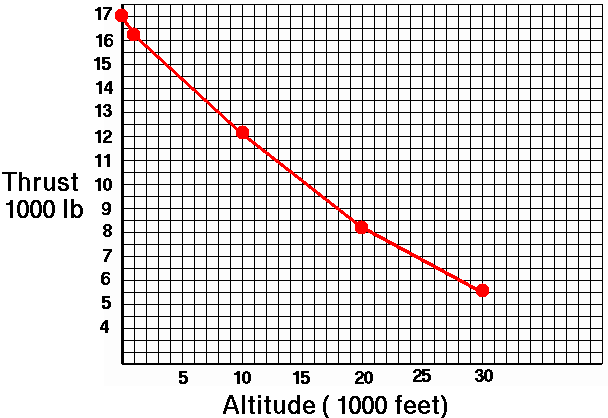19. Give your conclusions on thrust and flying at different altitudes.

Thrust will decrease with the change in higher altitude.

Related Pages:
Standards
Activity
Worksheet
Propulsion Activity Index
Propulsion Index+ Inspector General Hotline + Equal Employment Opportunity Data Posted Pursuant to the No Fear Act + Budgets, Strategic Plans and Accountability Reports + Freedom of Information Act + The President's Management Agenda + NASA Privacy Statement, Disclaimer, and Accessibility CertificationEditor: Tom Benson NASA Official: Tom Benson Last Updated: Thu, Jun 12 04:48:25 PM EDT 2014 + Contact Glenn